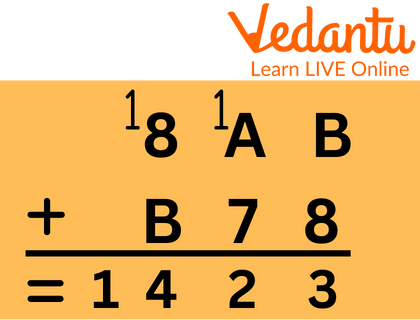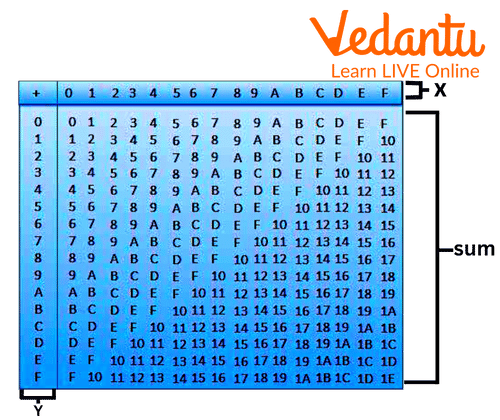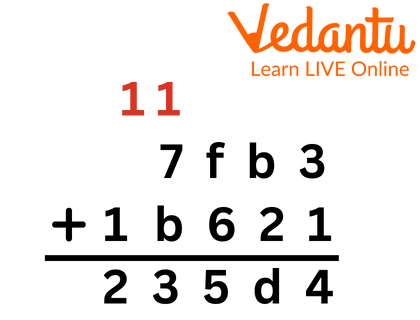Courses
Courses for Kids
Free study material
Free LIVE classes
MoreLIVE
Join Vedantu’s FREE Mastercalss

## An Overview of Hexadecimal Numbers

The letters "Hexa" and "deci" in the phrase "hexadecimal" stand for the numbers "6" and "10," respectively. The 16-digit hexadecimal representation of numerals 0 through 9 and letters A through F is known as the hexadecimal number system. To put it another way, the first nine numbers or digits are represented as numbers, while the next six digits are represented by the letters A through F. The decimal number system, which has a base of 9, and hexadecimal are quite similar. Therefore, after 9 digits, the 10th digit is represented as a symbol - 10 as A, 11 as B, 12 as C, 13 as D, 14 as E and 15 as F.

Since each digit has a weight of power 16, the hexadecimal number system is also known as a positional number system. Each subsequent digit is 16 times more important than the one before it. For example : $7B{3_{16}}$ ,$4B2{A_{16}},67{F_{16}}{\rm{ \;and }}{76_{16}}$

The only difference between hex addition and decimal addition is the addition of the letters A, B, C, D, E and F. Here is an illustration of hex addition. Check the example provided below, and for further information, see the paragraph below it.When numbers higher than 9 (the letters A through F) are present, hex addition includes calculating basic decimal addition while converting between hex and decimal. In the example, B + 8 equals 11 + 8 or 19.

You can perform hexadecimal addition much easier if you use the table below.The decimal number system, which has a base of 9, and the hexadecimal are quite similar. We can very easily add hexadecimal numbers by understanding the hexadecimal table and following simple addition.

Let’s understand it with the help of a example:Here, we will add from ones place:

So, 3 + 1 = 4

b + 2 = d

f + 6 = 15 (1will be in carry)

7 + b = 12

And as 1 was in carry over, so 12 + 1 = 13

Now, at last 1 + 1 = 2

Thus, the final answer is 235d4.

## Solved Examples

8AB + B78

Ans: 8AB + B78

We know:

8 + B = 13

A + 7 = 11

8 + B = 13

8AB + B78 = 1423

D6E + 8C6

Ans: We know that

E + 6 = 14

6 + C = 12

D + 8 = 15

Thus,

D6E + 8C6

= 1634

Therefore, its sum will be 1634.

Example 3: What use does the hexadecimal number system serve?

There is a way to write numbers in hexadecimal. Symbols are used when we write numbers. We continue adding digits and adding colour references until there are no more symbols left. Assembly language programming errors can also occur.

## Conclusion

The hexadecimal number system is also referred to as a positional number system since each digit has a power 16 value. The importance of each digit after the first one increases by a factor of 16.

Last updated date: 30th Sep 2023
Total views: 82.5k
Views today: 1.82k